# The Most Beautiful Equation of Math: Euler’s Identity

In 1988, a Mathematical Intelligencer poll voted Euler’s identity as the most beautiful feat of all of mathematics. In one mystical equation, Euler had merged the most amazing numbers of mathematics: $e^{i\pi}+1=0$.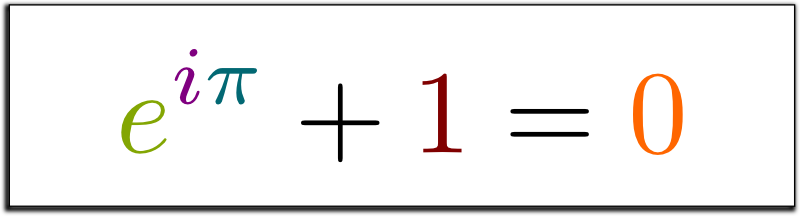What??? What are these symbols? What does that mean?

We’ll get there. But first, I want to insist one more time on the spectacular beauty of Euler’s identity. A recent study showed that Euler’s identity is so beautiful that it excites the same areas of the brains as a great piece of music or art would! If you don’t believe me, listen to the amazing William Dunham tell you about that:

I highly recommend you to watch the whole talk of William Dunham on Leonhard Euler’s achievements. It is one of the greatest talk I’ve heard watched!

## The Dream Team of Numbers

To fall in love with a story, you need to intimately know its characters. So, before talking about Euler’s identity, let’s draw portraits of the dream team of numbers that makes it up. Starting with the most important of them all, the $wau$ number, as presented below in this awesome video by ViHart:

The $wau$ number is amazing, because it usually is the solution of equations in such an obvious way that we even forget it is! For instance, $wau$ is the only solution to the equations $(x^2)! = x$ and $x^x = x$.

Wait… Do you mean that $wau = 1$?

Yes! And Historically, $wau$ is the first number invented, making it the father of all mathematics! For this mere reason, wouldn’t you agree to say that $wau$ is just such a more awesome name than 1?

Hahaha… I guess…

Let’s move on. Surprisingly, the second number to join the dream team is not $0$, but $\pi$. Yet, the number $\pi$ is actually a beautifully evasive number! $\pi$ first appeared in Antiquity, at least several millenia before our era, as the area of a disc of radius 1, or as the ratio of the circumference of a circle by its diameter. Thus, $\pi$ is the symbol of geometry.

So why do you say it is evasive?

Because it wasn’t until the 18th century that some basic properties of $\pi$ were discovered, like the fact that it is irrational. This means that $\pi$ cannot be written as the ratio of two numbers. A century later, it was then proved to even be transcendental, which means that $\pi$ is somehow beyond the realm of algebra.

Learn more about the relations between numbers and algebra in my articles on numbers and constructibility, and on Galois theory.

But, overall, the most important fact about $\pi$ is the fact that it has become a cultural symbol, as explained in this fun Math Bites with Danica McKellar on Nerdist:

While wau and $\pi$ are both ancient numbers, the other numbers of Euler’s identity are more recent. The number $0$ was only invented around the 7th Century in India. It is often attributed to Brahmagupta. And it deeply revolutionized our perspective on mathematics and numbers, as explained by the amazing Marcus du Sautoy in the BBC show Story of Math:

One important aspect of the invention of 0 was the natural extension of numbers to relative numbers. By then introducing negative numbers, the Indians developed the ideas of debts and investments, which were certainly a major and powerful economical innovation. In fact, 0 can be regarded as the father of algebra.

OK… But so far, I knew these numbers… What about $i$ and $e$?

The number $i$ is famously known as an imaginary number. Now, many would argue that this is a horribly wrong terminology, as $i$ occurs in so many areas that it is certainly not more imaginary as “real” numbers like $\pi$. And I agree. In fact, some modern mathematicians like Norman Wildberger argue that $i$ is way more “real” than $\pi$! In any case, the reason why I still find the terminology appealing is that the invention of $i$ has coincided with the (re)birth of pure mathematics.

What do you mean?

Historically, algebra was imported in Europe from the Muslim empire. But algebra wasn’t merely a powerful tool for engineers. It was also a perfect language to pose new abstract problems, like that of solving equations. And, amazingly, abstract equations like $x^2+1=0$ naturally led mathematicians to invent a number whose square would be $-1$. This number, sometimes abusively denoted $\sqrt{-1}$, is precisely $i$.

Learn more with my article on imaginary and complex numbers which constructs $i$ in a more natural way than the Historical one.
So, $i$ is just a piece of pure abstract mathematics with no connection to the real world?

At first, yes. Yet, surprisingly, notably because of Euler’s identity, the number $i$ now plays a central role in many fields, especially physics and engineering, as explained by the two following professors on Sixty Symbols:

Finally, last but not least, is Euler’s number $e$.

Did Euler merely invent it to write his identity?Not at all! The reason why Euler introduced $e$ was rather to describe the natural phenomenon of 100% continuous growths.

What do you mean?

Imagine you put 1 dollar in your bank, and they tell you’ll win a 100% interest rate every year. Now, because they are crooks, they’ll only compute your interest rate at the end of the year. Thus, you’ll get 1 more dollar, and obtain a total of 2 dollars.

Why did you say that they were crooks?

Because they waited for the end of the year to compute your gain! Instead, you may want to argue that the investment rate should be computed every month. Now, because it’s a 100% interest rate in twelve months, and because $100/12 \approx 8.33$, you should get an 8.33% rate every month. And if you do, amazingly, at the end of the year, instead of ending up with 2 dollars, you’ll have 2.61 dollars!

Waw! Indeed!

But wait! You can do even better by computing investment rates every day. Or even, every hour. Or every second. Eventually, by having a continuous growth, you would end up with $e \approx 2.718$ dollars at the end of the year. That’s how much you win a year with a 100% continuous growth.

If you want to know more about this intuitive approach to $e$ as 100% continuous growth, check this awesome article by Better Explained.

More formally, the 100% continuous growth means that $e$ satisfies the differential equation $e^x = d(e^x)/dx$, and our approach proves that $e = \lim (1+1/n)^n$. These facts make $e$ the symbol of calculus.

So there we go, we have our dream team of numbers!

Exactly! Let’s sum it up: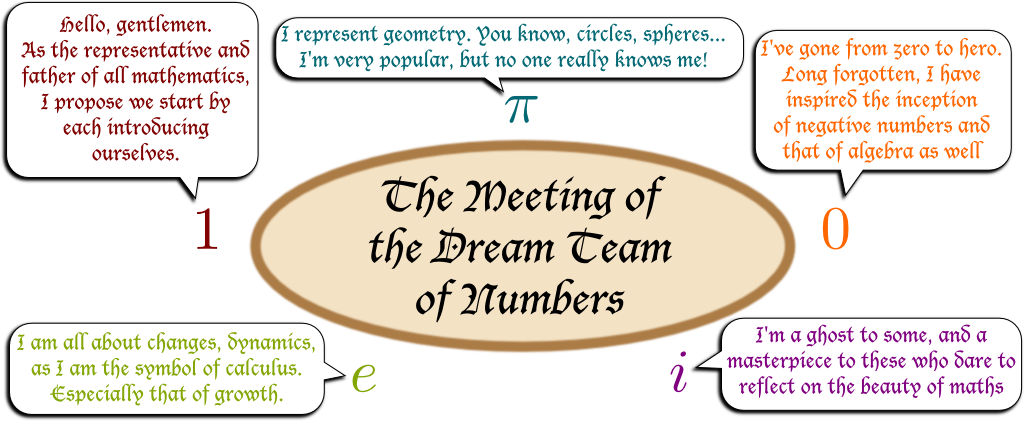Now, to think that all these guys, despite coming from diverse areas of mathematics, manage to merge together and form a breathtakingly neat equation… This really is mind-blowing! And it explains why Euler’s identity is so often regarded as the greatest feat of mathematics!

## The Intuitive Proof

Before getting to Euler’s original proof, let’s see the intuition underlying Euler’s identity. But to get there, I need to get you familiar with two major mathematical objects: The complex plane and derivatives.

This intuitive proof is very interesting because it involves some of the most important and beautiful elements of mathematics. It’s not that simple, but I strongly recommend you to try to fully understand it as it is extremely insightful.
What are these?

Let’s start with the complex plane, discovered by the giant Carl Friedrich Gauss a century after Euler’s time. Amazingly, while real numbers are points on a 1-dimensional line, imaginary and complex numbers perfectly correspond to points in a 2-dimensional plane. Indeed, a complex number can always be written uniquely $a+ib$, where $a$ and $b$ are the x and y-coordinates of the corresponding point.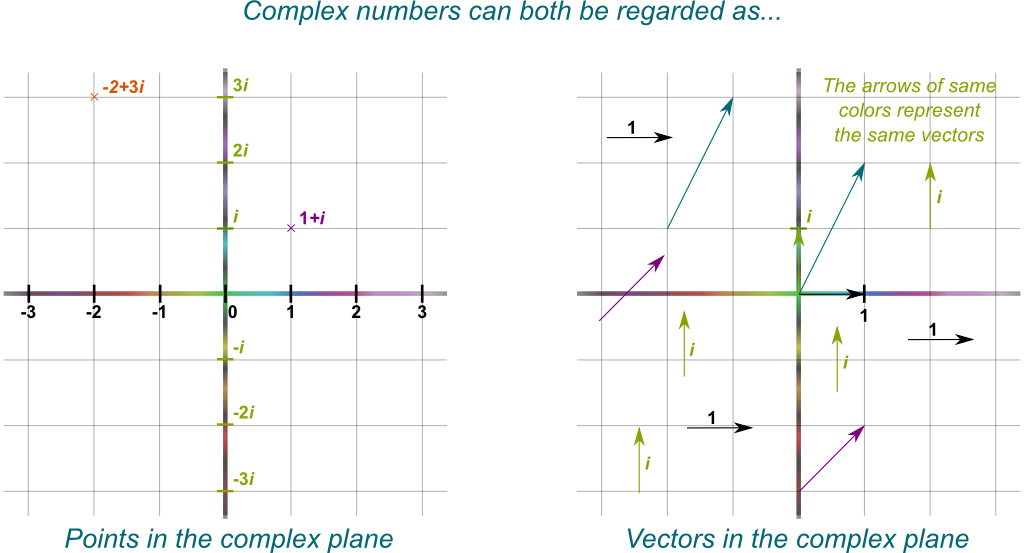A complex number can also be regarded as a motion, or vector, from the origin to the point it represents. This is important to understand the one last thing you need to know about complex numbers: Multiplying a complex number by $i$ corresponds to rotating the corresponding vector by an anti-clockwise quarter of turn. This will be essential in a bit…

Now, what are derivatives?

Derivatives describe motions, and they’re particularly visual when we consider trajectories in the complex plane.

What’s a trajectory in the complex plane?

A trajectory corresponds to a function $f$ which maps times $t$ to positions $f(t)$ in the complex plane. By varying $t$, the point $f(t)$ will be describing a trajectory in the complex plane. For instance, if $f(t) = t+it$, then $f$ will correspond to the trajectory that goes along the main diagonal at a constant velocity.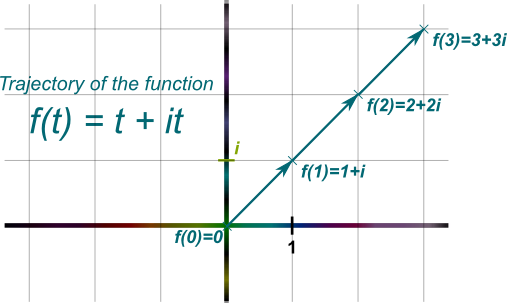So, really, a function which maps real numbers to complex numbers is just a trajectory in the complex plane.

OK… But what do these have to do with derivatives?

Following Newton’s footsteps, the derivative of a trajectory at a given time is its instantaneous velocity at that time. So, for instance, in the figure above, at any point, the instantaneous velocity is a motion going diagonally up-right. In fact, you may guess that it equals the vector $1+i$, as, in one unit of time $t$, the trajectory moves by $1+i$. Amazingly, this can also be deduced from basic laws of derivation.

What are these laws?

You’ve probably learned that the derivative of $\alpha t$ is $\alpha$ when $\alpha$ is a real number. Well, guess what, the same formula holds when $\alpha$ is a complex number! So, the derivative of $f(t) = t+it = (1+i)t$ is…

$1+i$. Amazing!

And now we’re getting closer and closer to proving Euler’s identity! As we have seen earlier, the fundamental property of Euler’s number $e$ is that the derivative of $e^t$ is $e^t$. Now, using the laws of derivation, it must be that the derivative of $e^{it}$ is $ie^{it}$.

The law I’m hinting at is the chain rule: The derivative of $u \circ v$ is $(u’ \circ v) v’$. These laws of differentiable should in fact be first generalized to all vector spaces. We could then show that it makes sense to consider that they hold for complex-valued functions.
Wait… Didn’t you say that multiplying by $i$ corresponds to a anti-clockwise vector rotation of a quarter of a turn?

Exactly! So, at a time $t$, the trajectory $e^{it}$ is at some point $e^{it}$ of the complex plane. Yet, $e^{it}$ is also the vector from the origin to $e^{it}$. Therefore, $ie^{it}$ is a vector perpendicular to the axis between the origin $e^{it}$ and 0, in the anti-clockwise direction. I know… it’s hard to read. So let me draw this: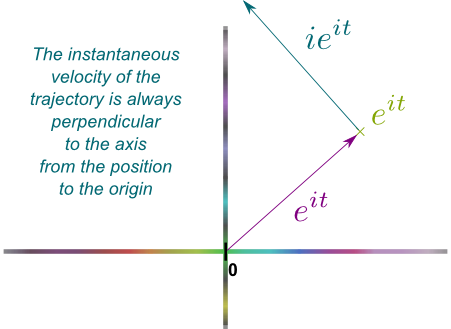Note how I used the notation $e^{it}$ to mean several different objects at once. There are the trajectory $e^{it}$ (which should more formally be denoted $t \mapsto e^{it}$), the point $e^{it}$ and the vector $e^{it}$. Similarly, there are the derivative $ie^{it}$ and the vector $ie^{it}$.

Now, if you have done some physics, you should immediately be able to figure out what kind of trajectory $e^{it}$ is.

I guess I haven’t done enough physics…

What if I told you that the origin was the Earth?

Then I guess that the point $e^{it}$ would correspond to the Moon…

Exactly! Just like the Moon, the velocity of the point $e^{it}$ always points perpendicularly to the axis Earth-Moon. And as a result…

I know! The trajectory of $e^{it}$ is that of a circle!

Bingo! The trajectory of $e^{it}$ is that of a circle rotating anti-clockwisely!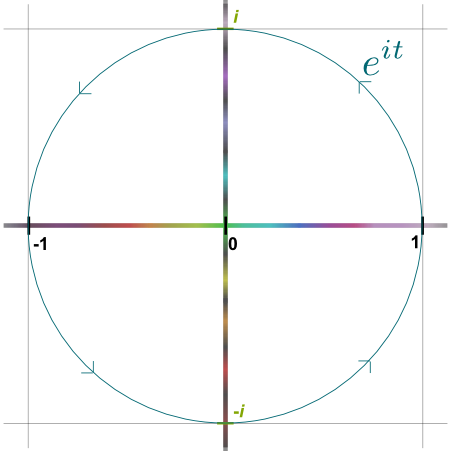You can prove this formally by studying the variation of the square of the distance between $e^{it}$ and the origin. Since this variation is 0, this means that the distance must be constant, and thus that $e^{it}$ must belong to a circle whose center is the origin.

Now, since $e^{it}=e^0 = 1$ if $t=0$, this means that this circle must go through 1. It’s thus necessarily the unit circle! Plus, since the velocity of the trajectory is the rotation of the vector $e^{it}$, the speed equals the length of the vector $e^{it}$, which is the radius of the circle: 1. Thus, the trajectory $e^{it}$ is a anti-clockwise rundown of the unit circle at speed 1. How beautiful is that?

Waw! This is so amazingly simple!

I know! And it enables to easily deduce the formula $e^{it} = cos(t) + i sin(t)$. But, we don’t even need that to derive Euler’s identity… What happens when $t=\pi$?

What do you mean?

Recall that at $t=0$, the trajectory $e^{it}$ started at 1. Now, it moves at speed 1 along the unit circle anti-clockwisely. So, at $t=\pi$, it will have walked a distance of $\pi$ on a circle of radius 1…

And since this circle has a circumference of $2 \pi$, it will have walked done half of a turn!

Exactly! This is where the geometry of the circle has $\pi$ entering in play! So, amazingly, $e^{i\pi}$ is the antipode of 1 on the unit circle, which is -1. Thus, $e^{i\pi}=-1$. Or, equivalently, we obtain the beautiful Euler identity:## Euler’s Proof

Now, this insightful and intuitive proof wasn’t Euler’s. Rather, Euler is known for his mastery of infinite sums, which he used to derive his identity.

This proof will not be as insightful as the previous one. However, it involves some other essential technics of mathematics. It is definitely more technical though.

The key objects of Euler’s proof are power series. A few decades before Euler, British mathematicians Brook Taylor and Colin Maclaurin found out that many real functions $f(t)$ could be written as an infinite sum of powers of $t$. In fact, in many cases, the derivatives of $f(t)$ at $t=0$ were sufficient to describe $f$ everywhere as such a sum. Denoting $f^{(n)}(0)$ the $n$-th derivative at $t=0$, the Taylor-Maclaurin series could then be written as follows: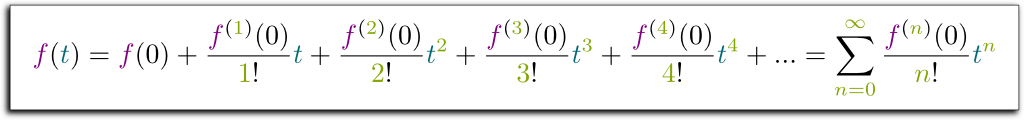Now, given that the derivative of $sin$ is $cos$, that the derivative of $cos$ is $-sin$, that $sin(0) = 0$ and that $cos(0) = 1$, we have:

• $sin(0)=0$, $sin^{(1)}(0)=cos(0)=1$, $sin^{(2)}(0)=-sin(0)=0$, $sin^{(3)}(0)=-cos(0)=-1$, $sin^{(4)}(0)=sin(0)=0$…
• $cos(0)=1$, $cos^{(1)}(0)=-sin(0)=0$, $cos^{(2)}(0)=-cos(0)=-1$, $cos^{(3)}(0)=sin(0)=0$, $cos^{(4)}(0)=cos(0)=1$…

Thus, the Taylor-Maclaurin series of the sine and cosine functions are the following ones: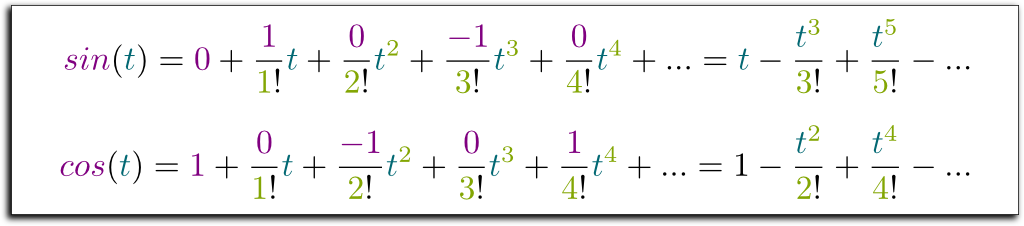Furthermore, we need to remark that if the derivative of $e^t$ is $e^t$ and if $e^0=1$, then, necessarily, all derivatives at all orders of $e^t$ in 0 must equal $e^0=1$. Thus, the Taylor-Maclaurin series of the $e^t$ is: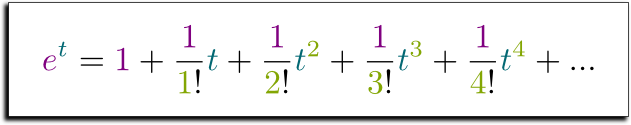Euler’s great move was to then replace $t$ by $it$ in the formula above. By then separating real parts from imaginary parts (I mean terms with $i$), magically, the expression of $e^{it}$ perfectly decomposes itself into $cos(t) + i sin(t)$. I’m a bit lazy so I won’t do that myself, but I’ll let this awesome video by VeritySeeker show it to you:

So, we have $e^{it} = cos(t) + i sin(t)$. By setting $t=\pi$, and given that $cos(\pi)=-1$ and $sin(\pi)=0$, we end up with $e^{it} = -1$. Or, equivalently,As you’ve guessed it, I’m not really fond of this proof, mostly because it’s a lot about computations. But also, it’s much trickier than it looks. First of all, we would need to define sine and cosine functions properly before. Then, we’d need to prove that the derivation properties of sine and cosine. Next, we’d still need to prove that $sin(\pi)=0$ and $cos(\pi)=-1$. Furthermore, we’d need to prove the Taylor-Maclaurin series of the sine, cosine and exponential functions indeed equal these functions, even when inputs are complex numbers. Eventually, all these tasks turn out to be longer and not easier than the intuitive proof we have given earlier (but historically, they were done, so it was easier for Euler to build upon them)… Finally, and that’s very important, our first proof was much more insightful.

## Let’s Sum Up

Euler’s identity is the greatest feat of mathematics because it merges in one beautiful relation all the most important numbers of mathematics. But that’s still a huge understatement, as it conceals a deeper connection between vastly different areas that Euler’s identity indicates. Above all else, Euler’s mystical identity is a clever insight into the perfection of the unit circle, by combining complex and diverse subfields of mathematics! In fact, the countless properties of this perfect unit circle are the reasons why Euler’s identity has become essential in many applications, like in physics.

Really?

Yes! And that’s strongly related to the unavoidable Fourier analysis. In essence, Fourier’s mind-blowing discovery is the fact that a large class of trajectories in the complex plane corresponds to infinite additions of unit circles run over at different speeds and in both directions. In calculus terms, this means that many functions $f(t)$ which map real to complex numbers can be written $\int g(\omega) e^{i\omega t} d\omega$, where $\omega$ is the speed of the rundown of unit circles. One important application of that is for the understanding of resonance.

Learn more with my article on Fourier analysis, and, for a more insightful but more abstract description of Fourier analysis, check my article on the dynamics of the wave function in quantum mechanics.

## More on Science4All

The Magic of AnalysisBy Lê Nguyên Hoang | Updated:2016-02 | Views: 1924
This article retraces the endless pursuit of the infinite that is at the basis of mathematical analysis. From the first approximations of pi to the shape of our limitless universe, from the essential usefulness of differential equations to the troubles with infinite sums, we present the great ideas of mathematical geniuses all along History.

Imaginary and Complex NumbersBy Lê Nguyên Hoang | Updated:2016-02 | Views: 6798
My first reaction to imaginary numbers was... What the hell is that? Even now, I have trouble getting my head around these mathematical objects. Fortunately, I have a secret weapon: Geometry! This article proposes constructing complex numbers with a very geometrical and intuitive approach, which is probably very different from what you've learned (or will learn).

Differential Calculus and the Geometry of DerivativesBy Lê Nguyên Hoang | Updated:2016-02 | Views: 7927
Differential calculus is one of the most important concept of mathematics for science and engineering. This article focuses on its fundamental meaning.

Construction and Definition of NumbersBy Lê Nguyên Hoang | Updated:2016-02 | Views: 9067
Although they have been used for thousands of years, an actual definition of numbers was given less than a century ago! From the most fundamental level of set theory, this article takes you to the journey of the construction of natural, integer, rational, real and complex numbers.

Logarithms and Age CountingBy Lê Nguyên Hoang | Updated:2015-12 | Views: 8590
Amusingly, the age difference between a 45-year-old man and a 25-year-old woman doesn't seem as big as the age difference between them 20 years earlier, when the woman was a little 5-year-old girl. This remark was the insight the late science popularizer Albert Jacquart liked to give to his readers to explain logarithms. This article pays tribute to the great scientist by introducing age difference as he liked to tell it.

The Revolutionary Galois TheoryBy Lê Nguyên Hoang | Updated:2016-02 | Prerequisites: Linear Algebra and Higher Dimensions, Imaginary and Complex Numbers, Symmetries and Group Theory | Views: 22109
In 1832, Évariste Galois died. He was 20. The night before his death, he wrote a legendary letter to his friend, in which he claims to have found a mathematical treasure! Sadly, this treasure had long been buried in total indifference! It took nearly a century to rediscover it! Since then, Galois' legacy has become some of the finest pure mathematics, which represents a hugely active field of research today with crucial applications to cryptography. Galois' work is now known as Galois theory. In essence, it unveils the hidden symmetries of numbers!

## More Elsewhere

1. I absolutely love your blog and find many of your post’s to
be just what I’m looking for. can you offer guest writers to write content for you personally?

I wouldn’t mind publishing a post or elaborating on many
of the subjects you write about here. Again, awesome website!

2.anal video says:

A round of applause for your blog.Thanks Again.

3.RosGerl says:

What s the deal with Euler s identity? Basically, it s an equation about numbers specifically, those elusive constants and

4.Sumit says:

hats off ! probably one of the best articles I have read.

5.Matt says:

This is an amazing post. Please keep writing!